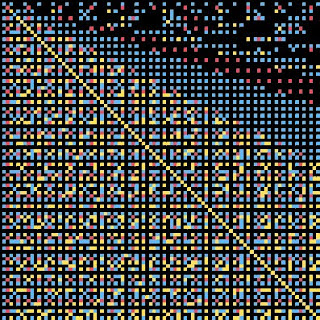## Wednesday, April 23, 2008

### Greatest Common Divisor of 3 Numbers

I have taken the coprimes and gcd project from two to three numbers. The gcd of three numbers can be computed as gcd(a, b, c) = gcd(gcd(a, b), c). When I use my coprimes or gcd project functions to plot examples, they tend to make the results a regular, checkerboard-like plot. Here's a example with the third number set to 6.I find this plot interesting because it takes the plot for coprimes, and forces a regular pattern into every six by six cell block.

Here's an example with the third number calculated, as row/col, rounded down to a whole number. It's interesting in that it appears the lower left is the basic coprimes pattern, which then fades out as it moves to upper right.Here's a larger version of the same plot, with slightly different color assignments.